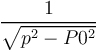# Discussion Forum

Discussion Closed This discussion was created more than 6 months ago and has been closed. To start a new discussion with a link back to this one, click here.

## Attempt to evaluate negative power of zero error

I am working on a COMSOL model of 2D axisymmetric packed bed for studying breakthrough profiles of gases. I have defined all my Overall balance, component balance, Ergun's equation and LDF model equation using Mathematical General form PDE model. I am facing the following error when I used the valve equation (w0) as a Constraint (w - w0) at the outlet of my bed, where w is the velocity in z direction. {Note:My model converged and gave the output when my constraint term was just w, meaning outlet of the bed is closed}

Feature: Time-Dependent Solver 1 (sol2/t1) Attempt to evaluate negative power of zero. - Function: ^ Failed to evaluate temporary symbolic derivative variable. - Variable: comp1.b@VDN$comp1.p - Defined as: comp1.y((comp1.p2)(0.5(((comp1.p^2)-(P0^2))^(-0.5)))) Failed to evaluate temporary symbolic derivative variable. - Variable: comp1.w0@VDN$comp1.p - Defined as: ((((TPstd)comp1.x)(comp1.y((comp1.p2)(0.5(((comp1.p^2)-(P0^2))^(-0.5))))))(1/(((comp1.peb0)A)Tstd)))+((Tstd(eb0A))((-(((TPstd)comp1.x)comp1.b))/((((comp1.peb0)A)Tstd)^2))) Failed to evaluate expression. - Expression: d(comp1.w-comp1.w0,comp1.p) Failed to evaluate Jacobian of expression. - Expression: comp1.w-comp1.w0

Questions:

1) How to define Modulus of a term and will that help to solve this problem? 2) What are the ways to solve this error, is there some kind of time stepping or meshing corrections to be made? Please suggest. Thanks

3 Replies Last Post 12 juni 2019 17:40 GMT−4

Posted: 1 year ago
Updated: 1 year ago

From your expression you are computingAnd apparently the numerator of this expression was identically 0 during your simulation. So the solution depends on these questions:

• Is the above expression correct?
• Is it physically possible for p and P0 to be equal in your case (or equal and opposite) ?
• if you answered yes to both of the questions above, what do you expect will happen physically when p= ± P0 ?

Posted: 1 year ago

I do not know the exact source of the problem in this case, but it is not uncommon that there is nothing suspicious in original expression, and that the problematic expression occurs only after internal symbolic differentiation. As soon as an expression contains a variable raised to a power <1, there is a risk of getting singular derivatives at zero.

In such cases, it often helps to add a small number (like the built-in variable ‘eps’) to the expression, so that the problematic denominator is never exactly zero.

Here is an example where the definition of a built-in variable within the field of soil plasticity is safeguarded in this way, since the square root is ‘dangerous’:

sqrt(solid.II2s+eps)+solid.lemm1.soil1.alpha*solid.I1s-solid.lemm1.soil1.k

Regards,
Henrik

-------------------
Henrik Sönnerlind
COMSOL